Mathematical and Physical Journal
for High Schools
Issued by the MATFUND Foundation
 Already signed up? New to KöMaL?

# KöMaL Problems in Physics, November 2019

Show/hide problems of signs:## Problems with sign 'M'

Deadline expired on December 10, 2019.

M. 390. By means of a prism made from water, in a simple way split the white light of a LED lamp into components. Write down the method and the result of the observation.

(6 pont)

statistics## Problems with sign 'G'

Deadline expired on December 10, 2019.

G. 685. The fuel tank of an American car can hold 15 gallons of gasoline. How many miles can the driver of the car go with the car, which was initially filled fully with gasoline, if according to the European catalogue of the car the fuel consumption of the car is 6.5 litres per 100 kilometres?

(3 pont)

solution (in Hungarian), statistics

G. 686. A 1.5-ton car is staying at rest on a horizontal road. Find the size of the surface the car touches the road, if the pressure in each tyre was adjusted to the value of 2.5 bars at the petrol station.

(3 pont)

solution (in Hungarian), statistics

G. 687. In a weather forecast not only the temperature but the feels like'' temperature is often given, which might be lower or higher than the temperature measured by the thermometer. When we have just finished a shower in the shower enclosure, then we feel the temperature warmer than the measured temperature in the bathroom, but if we open the door of the enclosure, because we left the towel outside, then our feels like'' temperature is much lower than the real temperature in the bathroom. Explain what is the reason for the fact that despite the temperature in the bathroom is nearly constant, our sense of temperature changes so abruptly.

(3 pont)

solution (in Hungarian), statistics

G. 688. Some time ago in the movies filmstrips, similar to the ones in children's tale film projectors, were used, just the movie filmstrips were much longer. The length of the filmstrip of a one-minute film was 27 metres. The films were wound on reels, which had a radius of 5.5 cm, and the width of the film roll on it was 12.5 cm. When a film was projected, the film was wound off the reel, called the feed reel, and wound on another reel, called the takeup reel.

$\displaystyle a)$ What was the number of revolution of the feed reel at the beginning and at the end of the projection of the film?

$\displaystyle b)$ After the projection the film was wound back from the takeup reel to the feed reel. What was the number of revolution of the takeup reel at the beginning and at the end of the re-wound process if the feed reel was rotated at constant 3 revolutions per second for all the time?

(4 pont)

solution (in Hungarian), statistics## Problems with sign 'P'

Deadline expired on December 10, 2019.

P. 5164. In the same amount of time, the number of complete small-amplitude swings of two simple pendulums are 5 and 10. What are the lengths of the pendulums if one of them is 120 cm longer than the other?

(3 pont)

solution (in Hungarian), statistics

P. 5165. Circles, which touch each other externally and whose centres are on the same radius of a uniform-density disc of unit radius, are cut out from the disc as shown in the figure.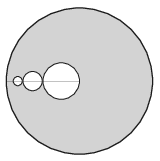The radii of the circles which are cut out are $\displaystyle \frac14, \frac18, \frac1{16}, \ldots\,.$ Where is the centre of mass of the remaining part of the disc if

$\displaystyle a)$ only the greatest circle,

$\displaystyle b)$ the two greatest circles,

$\displaystyle c)$ a lot of circles are cut out from the disc?

(5 pont)

solution (in Hungarian), statistics

P. 5166. A small object of 30 grams is attached to each end of a 40 cm long rod of an Eötvös pendulum. The extremely light rod is hanging on a very thin metal thread horizontally. A lead ball of mass 100 kg was placed to a distance of three metres from the centre of the rod at the same height as the rod.

$\displaystyle a)$ What is the torque exerted on the pendulum by the lead ball, when the angle between the line of the rod and the line which joins the centre of the rod and the ball is $\displaystyle \varphi$?

$\displaystyle b)$ Plot the torque as a function of the angle $\displaystyle \varphi$. At what angle will the torque be maximum?

(5 pont)

solution (in Hungarian), statistics

P. 5167. The faucet of a kitchen sink is opened slightly such that the water flows vertically at a constant rate and reaches the horizontal bottom of the sink. The diameter of the water flow at the bottom of the sink is $\displaystyle 3/4$ of the diameter of the water at 20 cm higher.

$\displaystyle a)$ What is the speed of the water at which it hits the bottom of the sink?

$\displaystyle b)$ What is the pressure exerted by the water at the bottom of the sink?

(5 pont)

solution (in Hungarian), statistics

P. 5168. In a closed cylinder there is a sample of helium gas of volume 4 dm$\displaystyle {}^3$. The base area of the cylinder is 7 dm$\displaystyle {}^2$ and the cylinder is closed with a 5 kg piston. The piston and the bottom of the cylinder is connected with a vertical, initially unstretched spring of spring constant 400 N/m.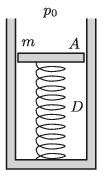How much heat should be added in order that the piston rise by 5 cm? (The external pressure is 100 kPa, and the system is thermally insulated.)

(4 pont)

solution (in Hungarian), statistics

P. 5169. One end of a uniform-density rod of mass $\displaystyle m=0.4$ kg and of length $\displaystyle L=20$ cm is attached to a hinge at point $\displaystyle A$, as shown in the left figure, about which it can be rotated in any direction. The other end of the rod is attached to a vertical spring of spring constant $\displaystyle D=25$ N/m, which also has a length of $\displaystyle L$ when there is no force exerted on it. Initially the spring and the rod are in the same line. Then the rod is displaced (as it is shown in the right figure) by an angle of $\displaystyle \varphi=60^\circ$, and then it is released.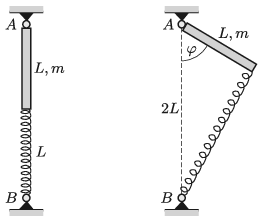$\displaystyle a)$ What is the speed of the end of the rod when it passes the vertical position?

$\displaystyle b)$ The rod is displaced from the equilibrium position by a small angle, and then it is released. How long does it take for the rod to reach the vertical position?

$\displaystyle c)$ By what angular speed should the spring-rod system be rotated about the vertical axis $\displaystyle AB$ in order that the angle between the rod and the vertical be constantly $\displaystyle 60^\circ$?

(Friction is negligible everywhere.)

(5 pont)

solution (in Hungarian), statistics

P. 5170. Some alike plastic straws were charged with rubbing and placed parallel to each other such that the line which joins their endpoints is perpendicular to the straws. It can be assumed that the distribution of charges on each straw is uniform, and all of them have the same charge. The two straws at the two ends are fixed such that their distance is much smaller than their length. Between them there are some straws which can move freely. What is the position of these straws if their number is

$\displaystyle a)$ two;

$\displaystyle b)$ three?

(5 pont)

solution (in Hungarian), statistics

P. 5171. An equilateral triangle is made of a piece of wire and two of its vertices are connected to a current supply as shown in the figure. The current in the wire which is connected to the vertex of the triangle is 10 A. What is the magnetic induction at the centre of the triangle? (The circuit is closed far from the triangle.)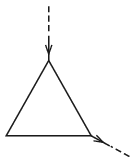(4 pont)

solution (in Hungarian), statistics

P. 5172. A shiny spoon is held in front of our eye at a distance of 25 cm such that the stem of the spoon is vertical. If the concave side of the spoon is observed, then an inverted image of our head can be seen, whilst if the convex part of the spoon is observed, then the image is upright. In which case will the height of the image of our head (its vertical size) appear greater? By what factor the angle subtended by the larger image is greater than that of the smaller image? The radius of curvature of the vertical section of the spoon is 5 cm.

(4 pont)

solution (in Hungarian), statistics

P. 5173. The core of a toroidal inductor of inductance $\displaystyle L=5$ H, number of turns $\displaystyle N=2000$, and of negligible ohmic resistance is a ring of high magnetic permeability. An ohmic resistor of resistance $\displaystyle R=200~\Omega$ is connected to the two terminals of the coil. A rechargeable battery of voltage $\displaystyle U_0=1{.}5$ V can be connected across one terminal of the coil and its 300th turn counted from that terminal.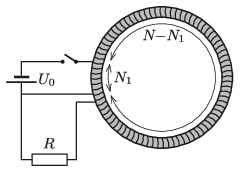$\displaystyle a)$ What is the current in the two parts of the coil $\displaystyle t_0=0{.}1$ s after the switch in the circuit was turned on?

$\displaystyle b)$ How much energy is supplied by the battery in the time of $\displaystyle t_0$, and what is this energy transferred to?

(6 pont)

solution (in Hungarian), statistics

P. 5174. In a lead container of an illegal laboratory some radiating material was found, which emits $\displaystyle 2\cdot 10^{14}$ electrons in a second. According to police reports 221 g caesium disappeared from the nearby research institute 53 years ago. Can the found material be the 53-year ago disappeared sample, if it was only stored? (The half life of caesium is 26.6 years.)

(4 pont)

solution (in Hungarian), statistics